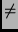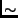Next: Accuracy Up: Round Robin Classification Previous: Class Binarization   Contents

Round Robin Classification

In this section, we will discuss a more complex class binarization procedure, the pairwise classifier. The basic idea is quite simple, namely to learn one classifier for each pair of classes. In analogy to round robin tournaments in sports and games, in which each participant is paired with each other participant, we call this procedure round robin binarization.1

Definition 3.1 (round robin/pairwise binarization)   The round robin or pairwise class binarization transforms a c-class problem into c(c-1)/2 two-class problems <i,j>, one for each set of classes {i,j}, i= 1 ... c-1, j = i+1 ...c. The binary classifier for problem <i,j> is trained with examples of classes i and j, whereas examples of classes ki,j are ignored for this problem.

12pt Round robin binarization is illustrated in Figure 1. For the 6-class problem shown in Figure 1(a), the round robin procedure learns 15 classifiers, one for each pair of classes. Figure 1(c) shows the classifier <+,>. In comparison, Figure 1(b) shows one of the classifiers for the unordered class binarization, namely the one that pairs class + against all other classes. It is obvious that in the round robin case, the base classifier uses fewer examples and thus has more freedom for fitting a decision boundary between the two classes. In fact, in our example, all binary classification problems of the round robin binarization could be solved with a simple linear discriminant, while neither the multi-class problem nor its unordered binarization have a linear solution. The phenomenon that pairwise decision boundaries can be considerably simpler than those originating from unordered binarization has also been observed in real-world domains. For example, Knerr et al. (1992) observed that the classes of a digit recognition task were pairwise linearly separable, while the corresponding one-against-all task was not amenable to single-layer networks. The results of Hsu and Lin (2002), who obtained a larger advantage of round robin binarization over unordered binarization for support vector machines with a linear kernel than for support vector machines with a non-linear kernel on several benchmark problems, could also be explained by simpler decision boundaries in the round robin case.

A crucial point, of course, is how to decode the predictions of the pairwise classifiers to a final prediction. We implemented a simple voting technique: when classifying a new example, each of the learned base classifiers determines to which of its two classes the example is more likely to belong to. The winner is assigned a point, and in the end, the algorithm will predict the class that has accumulated the most points. We break ties by preferring the class that is more frequent in the training set (or flipping a coin if the frequencies are equal). Note that some examples will be forced to be classified erroneously by some of the binary base classifiers because each classifier must label all examples as belonging to one of the two classes it was trained on. Consider the classifier shown in Figure 1(c): it will arbitrarily assign all examples of class o to either + or(depending on which side of the decision boundary they are). In principle, such ``unqualified'' votes may lead to an incorrect final classification. However, the votes of the five classifiers that contain examples of class o should be able to overrule the votes of the other ten classifiers, which pick one of their two constituent classes for each o example. If the class values are independent, it is unlikely that all classifiers would unanimously vote for a wrong class. However, the likelihood of such a situation could increase if there is some similarity between the correct class and some other class value (e.g., in problems with a hierarchical class structure). In any case, if the five o classifiers unanimously vote for o, no other class can accumulate five votes (because they lost their direct match against o). Nevertheless, this simple voting procedure is certainly suboptimal. We will discuss alternative decoding techniques in Section 7.

In the above definition, we assume that the problem of discriminating class i from class j is identical to the problem of discriminating class j from class i. This is the case if the base learner is class-symmetric. Rule learning algorithms, however, need not be class-symmetric. Many of them choose one of the two classes as the default class, and learn only rules to cover the other class. In such a case, <i,j> and <j,i> may be two different classification problems, if j is used as the default class in the former, and i in the latter. A straightforward approach for addressing this problem is to play a so-called double round robin, in which separate classifiers are learned for both problems, <i,j> and <j,i>.2

Definition 3.2 (double round robin)   The double round robin class binarization transforms a c-class problem into c(c-1) two-class problems <i,j>, one for each pair of classes (i,j), i,j = 1 ...c, ji. The examples of class i are used as the positive examples and the examples of class j as the negative examples.

24pt

In the following section, we will evaluate a double round robin as an alternative binarization strategy for the rule learner RIPPER.

 name train test sym num classes def. error abalone 3133 1044 1 7 29 83.5 car 1728 -- 6 0 4 30.0 covertype 15,120 565,892 44 10 7 51.1 glass 214 -- 0 9 7 64.5 image 2310 -- 0 19 7 85.7 letter 16,000 4000 0 16 26 95.9 lr spectrometer 531 -- 1 101 48 89.6 optical 5620 -- 0 64 10 89.8 page-blocks 5473 -- 0 10 5 10.2 sat 4435 2000 0 36 6 76.2 shuttle 43,500 14,500 0 9 7 21.4 solar flares (c) 1389 -- 10 0 8 15.7 solar flares (m) 1389 -- 10 0 6 4.9 soybean 683 -- 35 0 19 94.1 thyroid (hyper) 3772 -- 21 6 5 2.7 thyroid (hypo) 3772 -- 21 6 5 7.7 thyroid (repl.) 3772 -- 21 6 4 3.3 vehicle 846 -- 0 18 4 74.2 vowel 528 462 0 10 11 90.9 yeast 1484 -- 0 8 10 68.8Next: Accuracy Up: Round Robin Classification Previous: Class Binarization   Contents
Johannes Fürnkranz 2002-03-11